• 【OpenCV 例程200篇】04. 用 matplotlib 显示图像（plt.imshow
千次阅读
2021-11-05 19:14:34

## 【OpenCV 例程200篇】04. 用 matplotlib 显示图像（plt.imshow）

欢迎关注 『OpenCV 例程200篇』 系列，持续更新中
欢迎关注 『Python小白的OpenCV学习课』 系列，持续更新中

函数 plt.imshow() 用于通过 matplotlib 库显示图像。

函数说明：

matplotlib.pyplot.imshow(img[, cmap])

OpenCV 使用 BGR 格式，matplotlib/PyQt 使用 RGB 格式。使用 matplotlib/PyQt 显示 openCV 图像，要将 BGR 格式转换为 RGB 格式：

# 图片格式转换：BGR(OpenCV) -> RGB(PyQt5)
imgRGB = cv2.cvtColor(imgBGR, cv2.COLOR_BGR2RGB)


参数说明：

• img：图像数据，nparray 多维数组，对于 openCV（BGR）格式图像要先进行格式转换
• cmap：颜色图谱（colormap），默认为 RGB(A) 颜色空间
• gray：灰度显示
• hsv：hsv 颜色空间

注意事项：

1. OpenCV 和 matplotlib 中的彩色图像都是 Numpy 多维数组。但 OpenCV 使用 BGR 格式，颜色分量按照蓝/绿/红的次序排列，而 matplotlib 使用 RGB 格式，颜色分量按照红/绿/蓝的次序排序。因此用 plt.imshow() 显示 OpenCV 彩色图像时，先要进行颜色空间转换，将Numpy 多维数组按照红/绿/蓝的次序排序。
2. plt.imshow() 可以直接显示 OpenCV 灰度图像，不需要格式转换，但需要使用 cmap=‘gray’ 进行参数设置。
3. plt.imshow() 可以使用 matplotlib 库中的各种方法绘图，如标题、坐标轴、插值等，详见 matploblib Document
4. PyQt5 也使用 RGB 格式，因此在 PyQt5 中显示 OpenCV 彩色图像时，也要进行颜色空间转换。

基本例程：

    # 1.10 图像显示(plt.imshow)
imgFile = "../images/imgLena.tif"  # 读取文件的路径
img1 = cv2.imread(imgFile, flags=1)  # flags=1 读取彩色图像(BGR)

imgRGB = cv2.cvtColor(img1, cv2.COLOR_BGR2RGB)  # 图片格式转换：BGR(OpenCV) -> RGB(PyQt5)
img2 = cv2.cvtColor(img1, cv2.COLOR_BGR2GRAY)  # 图片格式转换：BGR(OpenCV) -> Gray

plt.rcParams['font.sans-serif'] = ['FangSong']  # 支持中文标签
plt.subplot(221), plt.title("1. RGB 格式(mpl)"), plt.axis('off')
plt.imshow(imgRGB)  # matplotlib 显示彩色图像(RGB格式)
plt.subplot(222), plt.title("2. BGR 格式(OpenCV)"), plt.axis('off')
plt.imshow(img1)    # matplotlib 显示彩色图像(BGR格式)
plt.subplot(223), plt.title("3. 设置 Gray 参数"), plt.axis('off')
plt.imshow(img2, cmap='gray')  # matplotlib 显示灰度图像，设置 Gray 参数
plt.subplot(224), plt.title("4. 未设置 Gray 参数"), plt.axis('off')
plt.imshow(img2)  # matplotlib 显示灰度图像，未设置 Gray 参数
plt.show()


程序说明：

图 1 中 OpenCV 的 BGR 彩色图像已转换为 RGB 格式，彩色图像的颜色显示正常；
图 2 中 OpenCV 的 BGR 彩色图像格式未做转换，彩色图像的颜色显示异常；
图 3 中 plt.imshow() 设置 cmap=‘gray’，灰度图像的颜色显示正常；
图 4 中 plt.imshow() 未设置 cmap=‘gray’，灰度图像的颜色显示异常。（本节完）

版权声明：

youcans@xupt 原创作品，转载必须标注原文链接

Crated：2021-11-18

欢迎关注 『OpenCV 例程200篇』 系列，持续更新中
欢迎关注 『Python小白的OpenCV学习课』 系列，持续更新中opencv python 图像处理 计算机视觉
更多相关内容
• 主要介绍了plt.imshow与cv2.imshow显示颜色问题,本文给大家介绍的非常详细，同时给大家提到了cv2.imshow()和plt.imshow()的区别讲解，需要的朋友可以参考下
• 主要介绍了解决python中显示图片的plt.imshow plt.show()内存泄漏问题，具有很好的参考价值，希望对大家有所帮助。一起跟随小编过来看看吧
• ## plt.imshow

千次阅读 2021-10-27 19:25:35
plt.imshow(X) X：图像数据 (M, N)：标量数据的图像，灰度图 (M, N, 3)：RGB图像 (M, N, 4)：RGBA图像 注意：其中RGB和RGBA图像为float类型[0, 1]，或者int类型[0, 255] 显示图像 Display an image, i.e. ...

## plt.imshow

plt.imshow(X, interpolation=None)

• X：图像数据

• (M, N)：标量数据的图像，灰度图
• (M, N, 3)：RGB图像
• (M, N, 4)：RGBA图像

注意：其中RGB和RGBA图像为float类型[0, 1]，或者int类型[0, 255]

显示图像

Display an image, i.e. data on a 2D regular raster.

import numpy as np
import matplotlib.pyplot as plt

np.random.seed(1)
# x = np.random.rand(25, 25)
x = np.random.rand(25, 25, 3)
# x = np.random.rand(25, 25, 4)

# 可以是float类型[0, 1]
print(x.dtype)
# plt.imshow(x)

# 也可以是int类型[0, 255]
max = np.max(x)
x = x*255/max  # expand pixel from [0, 1] to [0, 255], then normalization
# x = np.uint8(x) # 可以转化为uint8类型
x = x.astype(int) # 也可以转化为int32类型，都是为了使float-->int
print(x.dtype)
plt.imshow(x)
'''
输出：
float64
int32
'''


如果最后不显示图像的话，需要再加一句plt.show()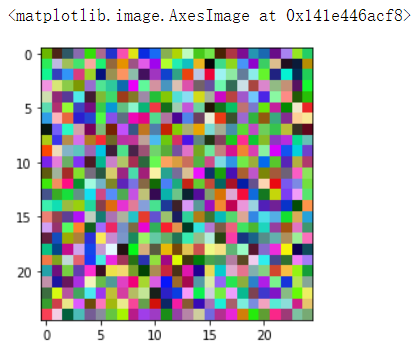### interpolation参数

这里特别讲一下interpolation参数，此参数显示了不同图像之间的插值方式

下面直接给出官方示例：链接

import matplotlib.pyplot as plt
import numpy as np

methods = [None, 'none', 'nearest', 'bilinear', 'bicubic', 'spline16',
'spline36', 'hanning', 'hamming', 'hermite', 'kaiser', 'quadric',
'catrom', 'gaussian', 'bessel', 'mitchell', 'sinc', 'lanczos']

# Fixing random state for reproducibility
np.random.seed(19680801)

grid = np.random.rand(4, 4)

fig, axs = plt.subplots(nrows=3, ncols=6, figsize=(9, 6),
subplot_kw={'xticks': [], 'yticks': []})

for ax, interp_method in zip(axs.flat, methods):
ax.imshow(grid, interpolation=interp_method, cmap='viridis')
ax.set_title(str(interp_method))

plt.tight_layout()
plt.show()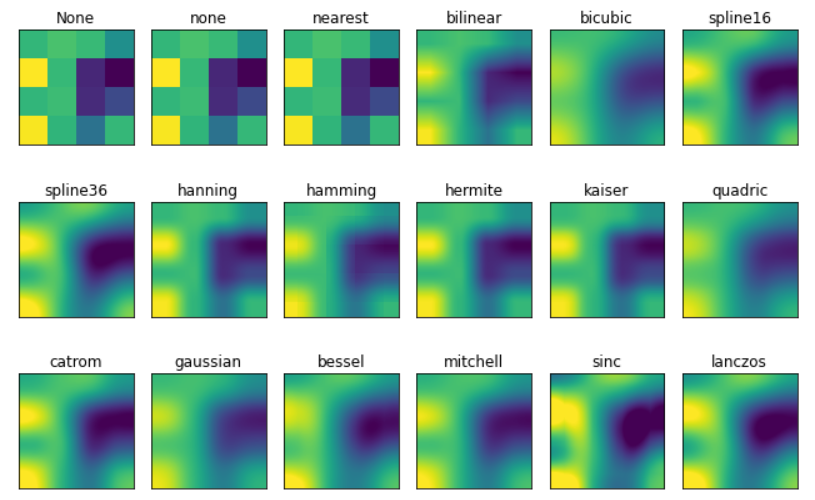展开全文计算机视觉 python opencv
• plt.imshow()将灰度图像进行彩色映射 CSDN 以下三张图（都是通过 matplotlib.pyplot.imshow 进行显示！！！） original 是原图(通过python的matplotlib.image.imread读取图像) RGB2BGR 是转换后的图(opencv图像操作...

以下三张图（都是通过 matplotlib.pyplot.imshow 进行显示！！！）

original 是原图(通过python的matplotlib.image.imread读取图像)
RGB2BGR 是转换后的图(opencv图像操作需要)，opencv使用BGR，matplotlib使用RGB，混用时显示会有差别
transforms_image 是初始化之后的图

初始化的函数：
transform = T.Compose(
[
T.ToPILImage(),
Resize(min_size, max_size),#调整图像尺寸[800~1333]
T.ToTensor(),#转tensor归一化到[0~1]
to_bgr_transform,
normalize_transform,#归一化到[-1~1]
]
)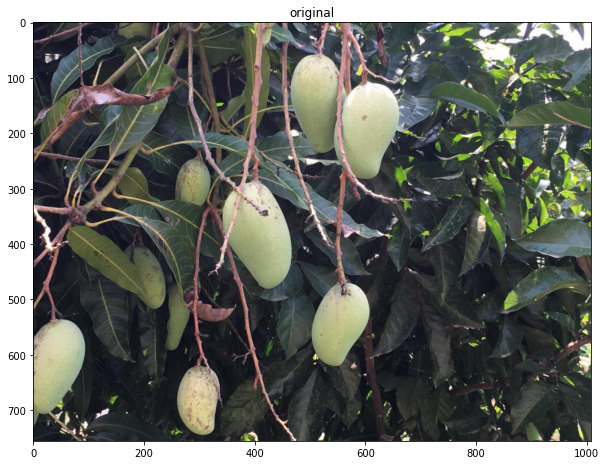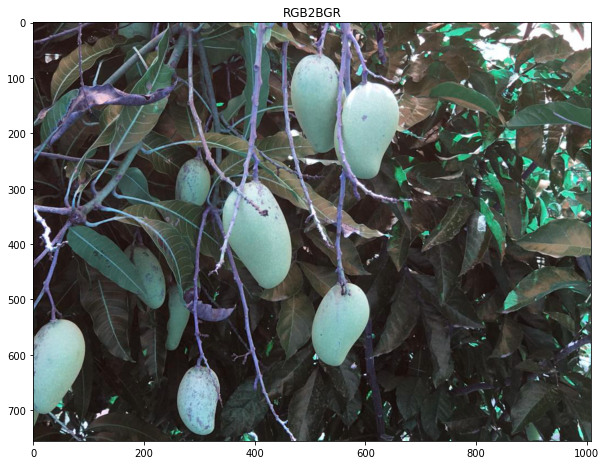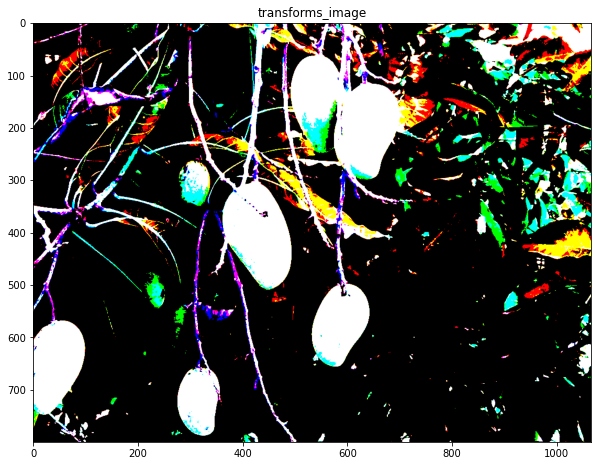这里主要讲一下transforms_image的图像的颜色问题，一个字：

'''
transforms_image 的打印结果
像素值有负数，我以为会显示不了，没想到也能显示，就离谱！
'''
tensor([[[ -61.9801,  -61.9801,  -62.9801,  ...,  121.0199,  105.0199,
92.0199],
[ -62.9801,  -62.9801,  -63.9801,  ...,  128.0199,  116.0199,
105.0199],
[ -62.9801,  -63.9801,  -63.9801,  ...,  138.0199,  130.0199,
123.0199],
...,
[ -50.9801,  -52.9801,  -54.9801,  ...,  -32.9801,  -27.9801,
-31.9801],
[ -55.9801,  -58.9801,  -62.9801,  ...,  -37.9801,  -31.9801,
-33.9801],
[ -64.9801,  -66.9801,  -69.9801,  ...,  -42.9801,  -34.9801,
-34.9801]],

[[ -90.9465,  -90.9465,  -91.9465,  ...,  131.0535,  119.0535,
111.0535],
[ -91.9465,  -91.9465,  -92.9465,  ...,  136.0535,  126.0535,
119.0535],
[ -91.9465,  -92.9465,  -92.9465,  ...,  139.0535,  136.0535,
130.0535],
...,
[ -66.9465,  -68.9465,  -70.9465,  ...,  -24.9465,  -17.9465,
-21.9465],
[ -71.9465,  -74.9465,  -78.9465,  ...,  -29.9465,  -21.9465,
-23.9465],
[ -80.9465,  -82.9465,  -85.9465,  ...,  -34.9465,  -24.9465,
-24.9465]],

[[ -96.7717,  -96.7717,  -97.7717,  ...,  120.2283,  108.2283,
99.2283],
[ -97.7717,  -97.7717,  -98.7717,  ...,  126.2283,  115.2283,
108.2283],
[ -97.7717,  -98.7717,  -98.7717,  ...,  130.2283,  125.2283,
120.2283],
...,
[ -81.7717,  -83.7717,  -85.7717,  ...,  -39.7717,  -32.7717,
-36.7717],
[ -86.7717,  -89.7717,  -93.7717,  ...,  -44.7717,  -36.7717,
-38.7717],
[ -95.7717,  -97.7717, -100.7717,  ...,  -49.7717,  -39.7717,
-39.7717]]])
Clipping input data to the valid range for imshow with RGB data ([0..1] for floats or [0..255] for integers).


于是我想着能不能把图像的颜色范围显示看一下，使用了plt.colorbar()函数，然后发现显示了个寂寞，图像中明明有黑色，在右边的 colorbar 上只有黄色到紫色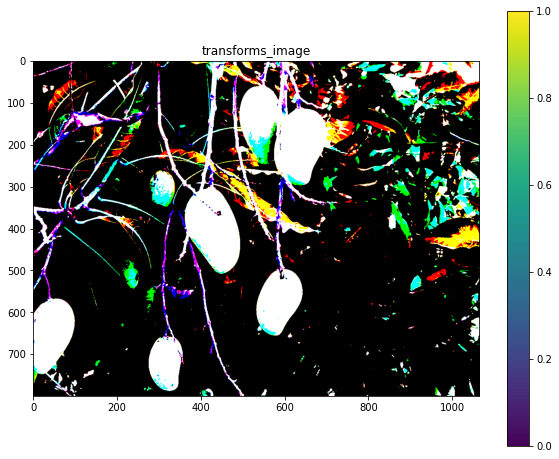后来看官方文档才了解到 matplotlib默认 的颜色范围就是黄到紫（也就是下面第二张图中的viridis颜色序列），这也就是问什么plt.imshow显示的二值图不是黑白两色而是是黄色和紫色两色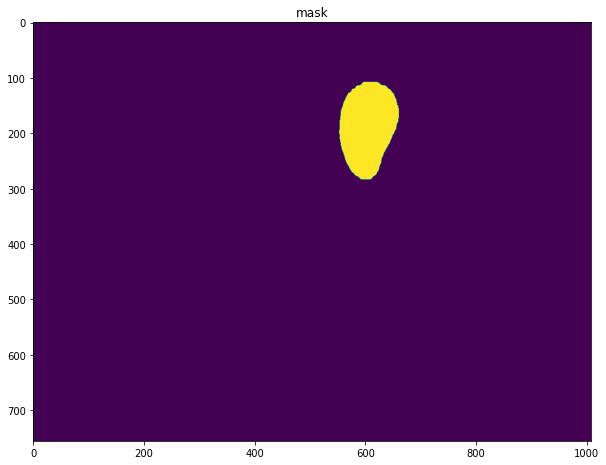设置了cmap参数：cmap='Greys' 的结果（真正的黑白二值图）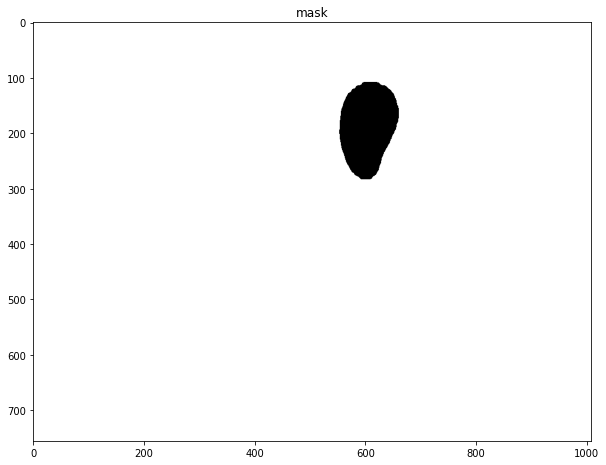如果想要使用其他的颜色序列可以使用cmap参数进行设置（下面有例子），最关键的是这个cmap参数只能对单通道图像有效，对多通道图像（RGB、RGBA）等无效，所以colorbar才会图像的颜色对不上

plt.title("transforms_image")
after_transforms = np.transpose(image, (1,2,0))#转换通道数
plt.imshow(after_transforms,cmap=plt.get_cmap('gist_gray'))#cmap='gist_gray'
plt.colorbar()
plt.show()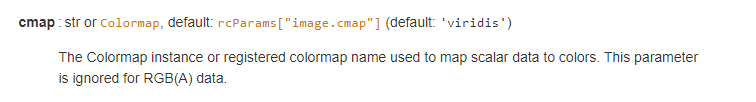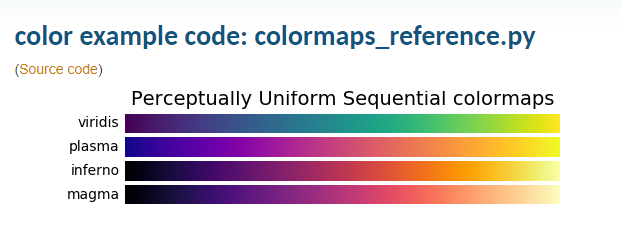下图是只显示单通道图像，设置了cmap=plt.get_cmap('gist_gray')之后的显示结果，这佐证了cmap参数只对单通道图像有效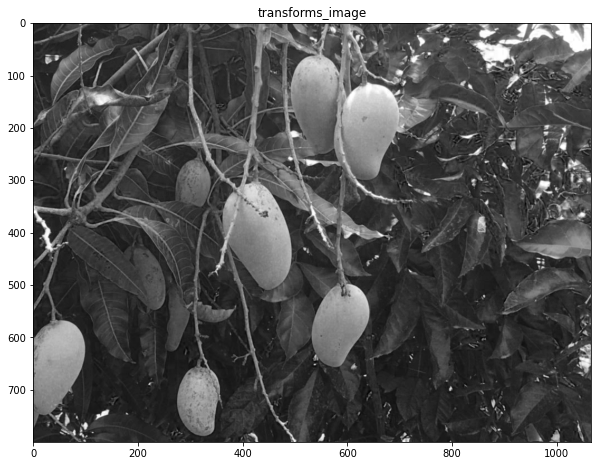最后，还是回到图像的像素值出现负数，plt.imshow怎么进行图像的显示的问题，其实官方文档已经进行了说明：只接受 [0~1][0~255] 范围内的像素值，超过得部分（包括负数）全部舍弃掉，从最后显示的结果来看，舍弃掉也就是用0像素值进行填充了，所以就出现了图像中的大部分的黑色区域，其他像素值正常的区域正常显示

注意（对超出范围的像素值进行舍弃的操作）只针对多通道图像（RGB、RGBA），单通道图像有负数也不会舍弃，它会按照数值的大小映射到颜色序列上（参考下面的例子像素值-100对应紫色，100对应黄色）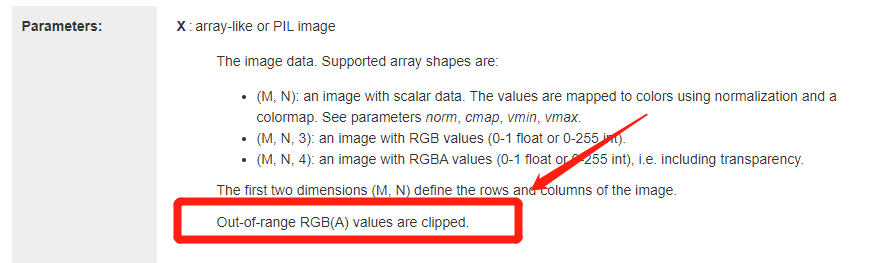'''多通道图像'''
ttt=np.zeros(shape=(100,100,3))
ttt[:,:,0]=np.linspace(-100,100,10000).reshape([100,100])
ttt[:,:,1]=np.linspace(-100,100,10000).reshape([100,100])
ttt[:,:,2]=np.linspace(-100,100,10000).reshape([100,100])
plt.figure(figsize=[10,8])
plt.title("ttt")
plt.imshow(ttt)
'''单通道图像'''
plt.figure(figsize=[10,8])
plt.title("ttt[:,:,0]")
plt.imshow(ttt[:,:,0])
plt.show()

ttt:
[[[-100.         -100.         -100.        ]
[ -99.979998    -99.979998    -99.979998  ]
[ -99.959996    -99.959996    -99.959996  ]
...
[ -98.05980598  -98.05980598  -98.05980598]
[ -98.03980398  -98.03980398  -98.03980398]
[ -98.01980198  -98.01980198  -98.01980198]]

[[ -97.99979998  -97.99979998  -97.99979998]
[ -97.97979798  -97.97979798  -97.97979798]
[ -97.95979598  -97.95979598  -97.95979598]
...
[ -96.05960596  -96.05960596  -96.05960596]
[ -96.03960396  -96.03960396  -96.03960396]
[ -96.01960196  -96.01960196  -96.01960196]]

[[ -95.99959996  -95.99959996  -95.99959996]
[ -95.97959796  -95.97959796  -95.97959796]
[ -95.95959596  -95.95959596  -95.95959596]
...
[ -94.05940594  -94.05940594  -94.05940594]
[ -94.03940394  -94.03940394  -94.03940394]
[ -94.01940194  -94.01940194  -94.01940194]]

...

[[  94.01940194   94.01940194   94.01940194]
[  94.03940394   94.03940394   94.03940394]
[  94.05940594   94.05940594   94.05940594]
...
[  95.95959596   95.95959596   95.95959596]
[  95.97959796   95.97959796   95.97959796]
[  95.99959996   95.99959996   95.99959996]]

[[  96.01960196   96.01960196   96.01960196]
[  96.03960396   96.03960396   96.03960396]
[  96.05960596   96.05960596   96.05960596]
...
[  97.95979598   97.95979598   97.95979598]
[  97.97979798   97.97979798   97.97979798]
[  97.99979998   97.99979998   97.99979998]]

[[  98.01980198   98.01980198   98.01980198]
[  98.03980398   98.03980398   98.03980398]
[  98.05980598   98.05980598   98.05980598]
...
[  99.959996     99.959996     99.959996  ]
[  99.979998     99.979998     99.979998  ]
[ 100.          100.          100.        ]]]

ttt[:,:,0]:
[[-100.          -99.979998    -99.959996   ...  -98.05980598
-98.03980398  -98.01980198]
[ -97.99979998  -97.97979798  -97.95979598 ...  -96.05960596
-96.03960396  -96.01960196]
[ -95.99959996  -95.97959796  -95.95959596 ...  -94.05940594
-94.03940394  -94.01940194]
...
[  94.01940194   94.03940394   94.05940594 ...   95.95959596
95.97959796   95.99959996]
[  96.01960196   96.03960396   96.05960596 ...   97.95979598
97.97979798   97.99979998]
[  98.01980198   98.03980398   98.05980598 ...   99.959996
99.979998    100.        ]]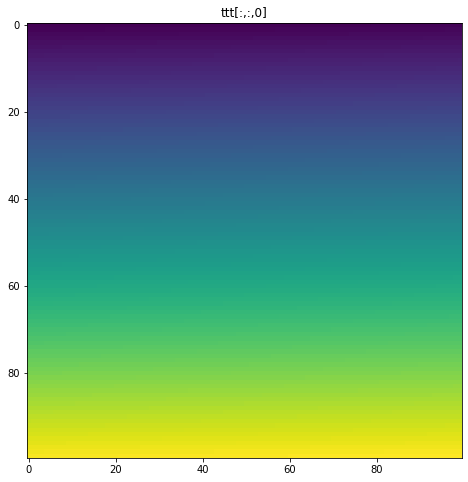展开全文opencv 计算机视觉 matplotlib
• 最近在学习基于python的数字图像处理，遇到了如标题中的问题。 不明白为啥有时候是plt.imshow有时是io.imshow 顺便问一下imshow函数和io类的具体作用，网上的解释不是很详细有问必答 python
• imshow()其实就是将数组的值以图片的形式展示出来,数组的值对应着不同的颜色深浅,而数值的横纵坐标就是数组的索引,比如一个1000X1000的数组,图片里的点也就有1000X1000个,比如第一个行第一个点的坐标就是(0,0),它的...

imshow()其实就是将数组的值以图片的形式展示出来,数组的值对应着不同的颜色深浅,而数值的横纵坐标就是数组的索引,比如一个1000X1000的数组,图片里的点也就有1000X1000个,比如第一个行第一个点的坐标就是(0,0),它的值会通过colorbar(也就是cmap)反映出来,所以按照我的理解，imshow()函数的功能就是把数值展示成热图。下面是一个简单的代码段：

x = np.linspace(0, 10, 1000)
I = np.sin(x) * np.cos(x[:, np.newaxis])
plt.imshow(I, cmap='RdBu')
cb = plt.colorbar(label='color bar settings')
plt.show()


而整个imshow()函数中的数组的值,则可以通过plt.colorbar()来展示。之后会在右边出现一条颜色bar，而bar的刻度范围（默认情况下）对应的正是数组的最大值到最小值的范围。

x = np.linspace(0, 10, 1000)
I = np.sin(x) * np.cos(x[:, np.newaxis])
speckles = (np.random.random(I.shape) < 0.01)
I[speckles] = np.random.normal(0, 3, np.count_nonzero(speckles))
plt.figure(figsize=(10, 3.5))

plt.subplot(121)
plt.imshow(I, cmap='RdBu')
plt.colorbar(label='noisy points')

plt.subplot(122)
plt.imshow(I, cmap='RdBu')
plt.colorbar(extend='both', label='noisy points extend')
plt.clim(-1, 1)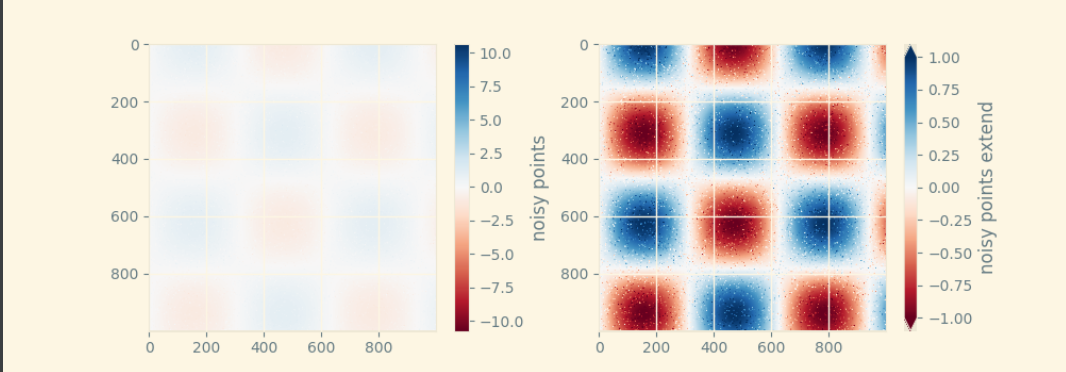上面这段代码绘制了两幅图片，一副图是默认的colorbar刻度范围（左图），包含了数组的最大值和最小值；另一幅则自己选定了展示的范围，plt.clim(-1,1)将展示的数据范围锁定在(-1,1)之间（右图）。其余的超出范围的值则通过设置extend参数，使其展示为噪点，extend='both'就是colorbar上下都会有两个小三角。通过下面这个在console中调试的代码段我们可以发现，colorbar的范围确实是数组的值的范围。

print(np.max(I))
11.73080564750175
print(np.min(I))
-12.962268087002979

那为什么第一张图的颜色被冲淡了呢？

我通过改变数组的最大值和最小值，想做做对比：

I[3, 2] = 30
plt.imshow(I, cmap="RdBu")
plt.colorbar(label='max value test')
plt.show()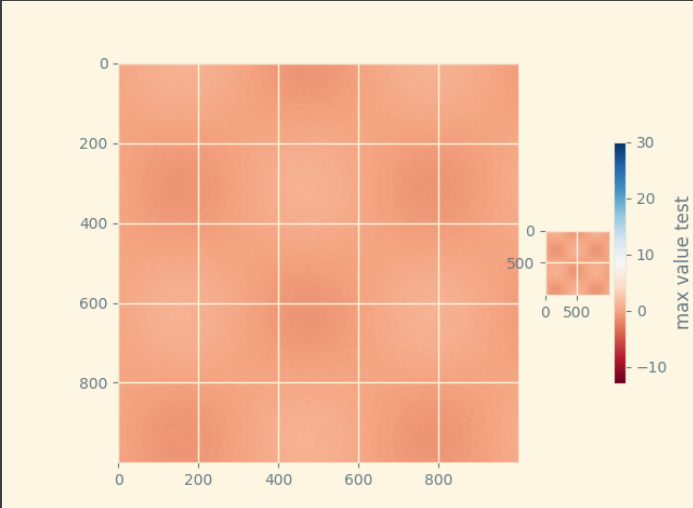这是改变了最大值时，可以看出整个图片集中在暖色区，这是因为最大值设置之后，整个颜色条的颜色上移了，在（-1,1）范围内的颜色都是暖色调的，而原本数组中的数值大部分也都在整个范围内，所以展示出来的颜色图也都是暖色；

I[2, 3] = 30
I[10, 20] = -200
plt.imshow(I, cmap="RdBu")
plt.colorbar(label='min and max value test')
plt.show()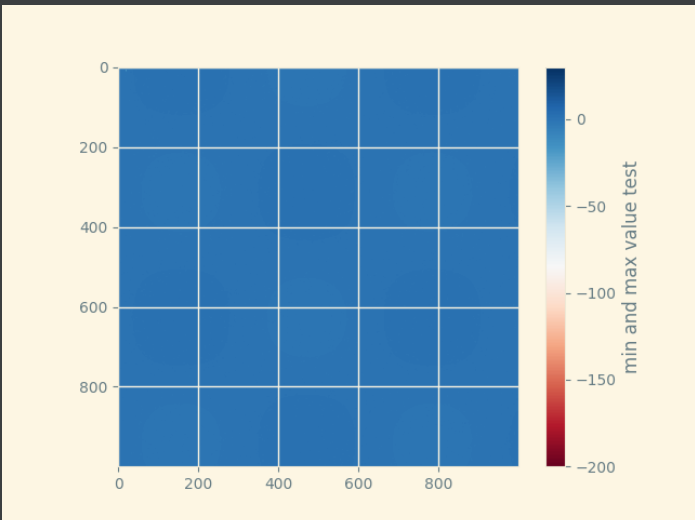而当我们把最小值设置成200的时候，整个颜色bar下移了，在（-1,1）范围内的值的颜色大多是冷色调，因此展示出来的色图也就看起来是一片蓝色。

综上，最开始的两张图中的左图，是因为有几个个别的点超出了（-1,1）的范围，导致色块的范围变大，但实际上许多色块区域的颜色并没有出现在图中，也就是没有点与其对应，而大部分点落在的（-1,1）范围内的颜色都是浅蓝色（正数）和浅红色（负数），因此整个色图颜色比较浅，也就是被几个噪点冲淡了；但是当我们设置参数后，色块范围变成了（-1,1），这样大量的点也都在这个范围内，每个点对应的颜色也就区分开来，色彩比较丰富，深浅比较明晰，就是右图所示，更加清晰可见的展示了负数中心和正数中心。而图上的许多小点点就是超出色块范围的噪点。

最后，extend参数如果不设置的话，唯一的影响就是右边的colorbar没有上下两个小三角显示出来。（目前看来是这样的）

展开全文python
• 文章目录一、图像红变蓝，蓝变红的问题（1）原因分析（2）代码及结果展示1）错误代码2）错误结果3）正确代码二、深度学习数据包plt.imshow绘制图像偏蓝黄色（1）原因分析1）原理解释代码2）结果（2）实际绘图分析与...
• plt.imshow显示cv2读取的图像颜色不对的解决方案 (plt.imshow与cv2.imshow显示同一图像颜色不一致) 原理 Opencv: cv2.imread( ) 所读取的图像格式：每个像素为[B,G,R]的形式 cv2.imshow( ) 显示图像：按图像中每个...计算机视觉 opencv python
• plt.imshow或者cv2.imshow时候，如果不设置显示停留，则图像会一闪而过。 解决方案： #matplotlib.pyplot plt.show() #opencv2 cv2.waitKey(0) 示例代码： img = cv2.imread('..png') rows, cols = img....
• import matplotlib.pyplot as plt 祖传代码，解决图中文显示问题 import matplotlib as mpl # 排除警告信息 import warnings # matplotlib画图常见参数设置 mpl.rcParams["font.family"] = "SimHei" # 设置字...opencv 计算机视觉 图像识别
• 今天遇到了一个Bug，花了很大的时间精力解决，在这里记录一下。 Bug如题目所说，在对一张渲染出来的图片保存时，发现存成了全黑... plt.imshow(rendering) plt.show() plt.axis('off') 使用cv2.imshow显示正常.图像处理
•python
• 在运行了以下程序后不显示图像： train_set_x_orig, train_set_...在后面加上plt.show()就会显示图像了 ，plt.imshow()函数对图像进行处理，并显示其格式。plt.show()则是显示plt.imshow()处理后的函数。 train_setpython
• plt.imshow() 显示全部都为空白 1、 错误提示 在使用 matplotlib 绘制图片时显示空白，且提示： Clipping input data to the valid range for imshow with RGB data ([0..1] for floats or [0..255] for ...matplotlib python
• 解决使用pyhon OpenCv傅里叶逆变换后plt.imshow( )能正常显示，而cv2.imshow( )显示为白色和保存图像为白色的问题。opencv python 计算机视觉 图像处理
• plt.show()之后，程序会暂停到那儿，并不会继续执行下去。如果需要继续执行程序，就要关闭图片。 如何展示动态图或多个窗口呢？ 使用plt.ion()这个函数，使matplotlib的显示模式转换为交互（interactive）模式。...
• 本篇文章小编给大家分享一下python中plt.imshow与cv2.imshow显示颜色问题代码解析，文章介绍的很详细，小编觉得挺不错的，现在分享给大家供大家参考，有需要的小伙伴们可以来看看。在用plt.imshow和cv2.imshow显示同...
• ## plt.imshow()

万次阅读 2018-11-15 21:25:00
plt.imshow(X) plt.colorbar() plt.show() 运行结果如下图所示，其中左上角颜色为蓝色，对应值为1；右下角颜色为深红色，对应值为6。它是按照矩阵X进行颜色分布的。 [1, 2] [深蓝, 浅蓝] [3, 4] [淡绿, 黄色] [5, ...
• plt.imshow( ) 不会转换通道， cv2.imwrite( ) 将通道逆序python
• 在用plt.imshow和cv2.imshow显示同一幅图时可能会出现颜色差别很大的现象。 这是因为：opencv的接口使用BGR，而matplotlib.pyplot 则是RGB模式。 各自显示各自图像是正常的，分别显示是不正常的。 说起来很绕，还是...python 图像处理 pycharm 计算机视觉...